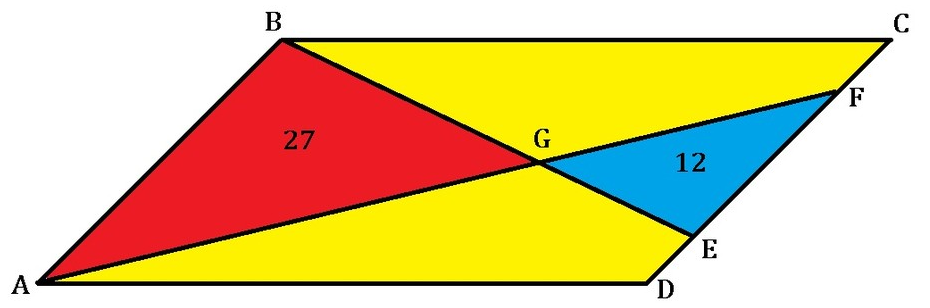# Parallelogram Riddle IIAs shown above, a parallelogram $ABCD$ is partitioned by two lines $AF$ and $BE$, such that the areas of the red $\triangle ABG = 27$ and the blue $\triangle EFG = 12$.

What is the area of the yellow region?

Note: Figure not drawn to scale.

×